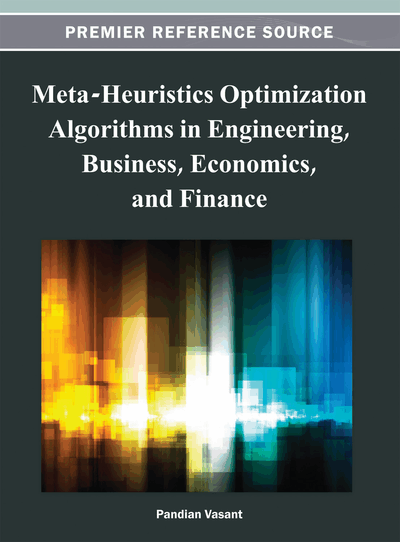# Hybrid Linear Search, Genetic Algorithms, and Simulated Annealing for Fuzzy Non-Linear Industrial Production Planning Problems

P. Vasant (University Technology Petronas, Malaysia)
DOI: 10.4018/978-1-4666-2086-5.ch003
Available
\$37.50
No Current Special Offers

## Abstract

This chapter outlines an introduction to real-world industrial problem for product-mix selection involving eight variables and twenty one constraints with fuzzy technological coefficients, and thereafter, a formulation for an optimization approach to solve the problem. This problem occurs in production planning in which a decision maker plays a pivotal role in making decision under fuzzy environment. Decision-maker should be aware of his/her level of satisfaction as well as degree of fuzziness while making the product-mix decision. Thus, a thorough analysis is performed on a modified S-curve membership function for the fuzziness patterns and fuzzy sensitivity solution is found from the various optimization methodologies. An evolutionary algorithm is proposed to capture the optimal solutions respect to the vagueness factor and level of satisfaction. The near global optimal solution for objective function is obtained by hybrid meta-heuristics optimization algorithms such as line search, genetic algorithms, and simulated annealing.
Chapter Preview
Top

## Introduction

It is well known that optimization problems arise in a variety of situations. Particular interesting are those concerning management problems as decision makers who usually state their data in a vague way such as “high profits”, “low cost”, “average revenue”, etc. Because of this vagueness, managers prefer to have not just one solution but a set of them so that the most suitable solution can be applied according to the state of existing decision of the production process at a given time without increasing delay. In these situations, fuzzy optimization is an ideal methodology since it allows us to represent the underlying uncertainty of the optimization problem while finding optimal solutions that reflect such uncertainty. Once the uncertainty has been solved, the obtained solutions are then applied to possible instances. This allows us to obtain a model of the behavior of the solutions based on the uncertainty of the optimization problem.

Fuzzy constrained optimization problems have been extensively studied since the years of seventies. In the linear case, the first approaches to solve the so-called fuzzy linear programming problem appeared in Bellman and Zadeh (1970). Since then, important contributions for solving different linear models have been made and these models have been the subject of a substantial amount of work. In the nonlinear case (Ramik and Vlach, 2002) the situation is quite different, as there is a wide variety of specific and both practically and theoretically relevant nonlinear problems with each having a different solution method.

In this chapter, a real-life industrial problem for product mix selection involving 21 constraints and eight bound constraints has been considered. This problem occurs in production planning, in which a decision-maker plays a pivotal role in making decision under a highly fuzzy environment (Vasant, Bhattacharya, Sarkar and Mukherjee, 2007; Vasant, Barsoum, Kahraman and Dimirovski, 2007). Decision maker should be aware of his/her level-of satisfaction as well as the degree of fuzziness while making the product mix decision. Thus, we have analyzed the problem using the sigmoidal membership function, fuzziness patterns and fuzzy sensitivity of the solution. Vasant (2006) considered a linear case of the problem and solved it by using a linear programming iterative method which is repeatedly applied for different degrees of satisfaction values. In this research, a non linear case of the problem is considered and a various optimization approaches are proposed in order to capture solutions for different levels of satisfaction with a single and multiple run of the algorithm. These various optimization approaches have been proposed by Liang (2008), Sanchez, Jimenez and Vasant (2007), Turabieh, Sheta and Vasant (2007), Bhattacharya, Abraham, Vasant and Grosan (2007), Bhattacharya, Vasant, Sarkar and Mukherjee (2006) and Jim´enez, G´omez-Skarmeta and S´anchez (2004) within a soft computing optimization general context. The detail on the nonlinear case study will be provided in the following section.

### Line Search (LS)

In this chapter, the line search method in solving industrial production planning problems is focused. The main advantage of this method is its ability to locate the near global optimal solutions for the fitness function with its strong criteria of global convergence. The line search method used fmincon approach from MATLAB computational toolbox. FMINCON is a gradient-based method that has been designed to work on problems where the objective and constraint functions are both continuous and have continuous first derivatives. The function with continuous first and second derivatives is suitable for the optimization process because the algorithm uses gradient-based methods. FMINCON uses a sequential quadratic programming (SQP) method. In this method, the function solves a quadratic programming (QP) of sub-problem at each iteration. A line search is performed using a merit function similar to that proposed by Powel (1983). The QP sub-problem is solved using an active set strategy similar to that described in (Powel, 1983). A full description of this algorithm can be found in Constrained Optimization in Standard Algorithms (Powel, 1983).

## Complete Chapter List

Search this Book:
Reset